Auto ByteScience AI# 飞桨实战笔记：自编写模型如何在服务器和移动端部署

SSD模型介绍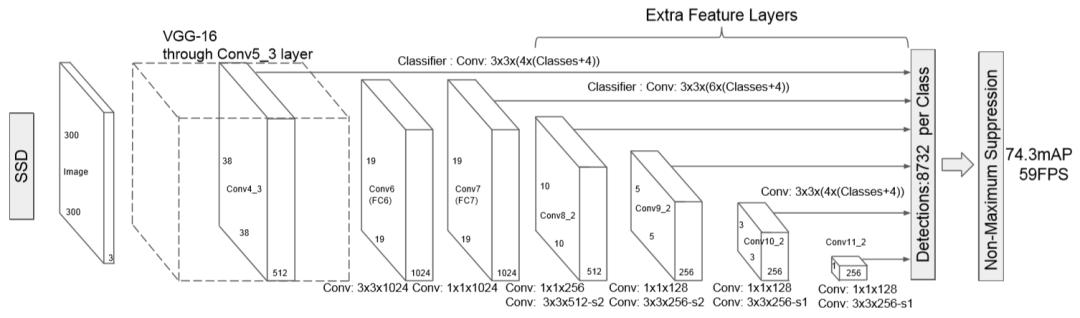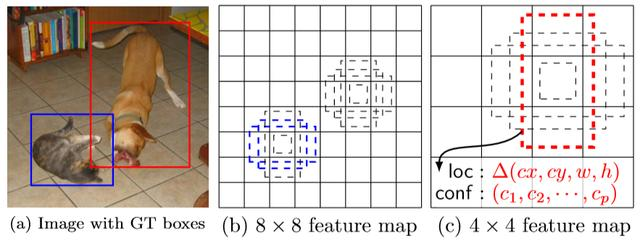b图就是我们以每个网格为中心，取到的先验框的示例。c图的回归预测找到了目标的位置信息，分类预测确定了物体的类别。a图代表了最终的结果。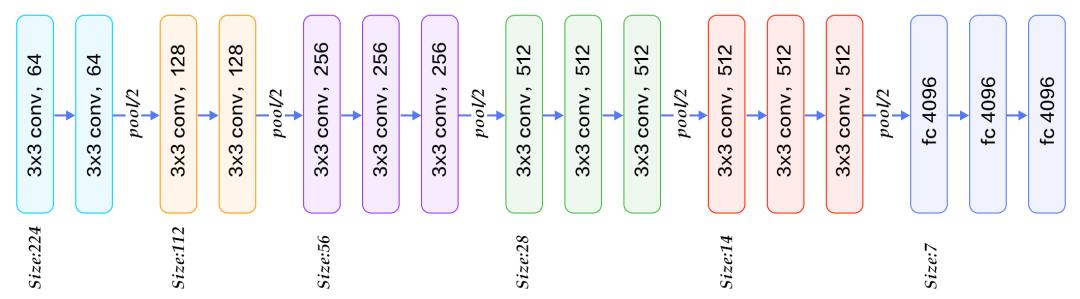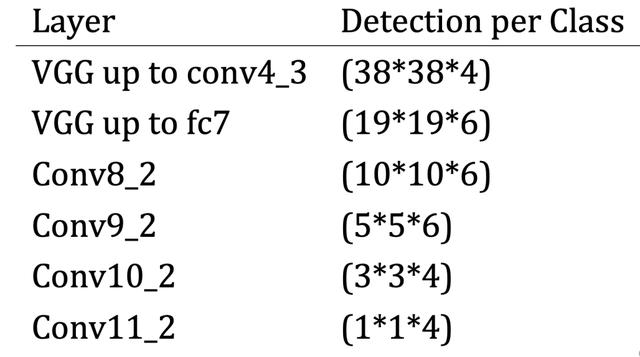MobileNet 与 SSD结合

MobileNet将卷积分为Depthwise和Pointwise两部分，减少了计算量，同时不会损失过多的精度。也因此在移动设备和嵌入式设备上面有很好的应用前景。更多关于MobileNet的理论信息大家可以在网上找到，这里不做过多讲述。```# Run CPU Docker docker pull hub.baidubce.com/paddlepaddle/serving:0.2.0 docker run -p 9292:9292 --name test -dit hub.baidubce.com/paddlepaddle/serving:0.2.0 docker exec -it test bash

`python3 -m pip install paddle_serving_server sentencepiece opencv-python pillow -i https://pypi.tuna.tsinghua.edu.cn/simple`

`import paddle_serving_client.io as serving_io serving_io.save_model(                     "ssd_model",                     "ssd_client_conf",                     {'image': img},                     {"prediction": box},                     inference_program)`

```img: name: "img" type {   type: LOD_TENSOR   lod_tensor {     tensor {       data_type: FP32       dims: -1       dims: 3       dims: 300       dims: 300     }     lod_level: 0   } }
persistable: false box: name: "concat_0.tmp_0" type {   type: LOD_TENSOR   lod_tensor {     tensor {       data_type: FP32       dims: 1917       dims: 4     }     lod_level: 0   } }
persistable: false```

```import os import sys import base64 import numpy as np import importlib from paddle_serving_app import ImageReader from multiprocessing import freeze_support from paddle_serving_server.web_service import WebService

class ImageService(WebService):     def preprocess(self, feed={}, fetch=[]):         reader = ImageReader(image_shape=[3, 300, 300],                              image_mean=[0.5, 0.5, 0.5],                              image_std=[0.5, 0.5, 0.5])         feed_batch = []         for ins in feed:             if "image" not in ins:                 raise ("feed data error!")             sample = base64.b64decode(ins["image"])             img = reader.process_image(sample)             feed_batch.append({"image": img})         return feed_batch, fetch

```import requests import base64 import json import time import os import sys
py_version = sys.version_info

def predict(image_path, server):     if py_version == 2:         image = base64.b64encode(open(image_path).read())     else:         image = base64.b64encode(open(image_path, "rb").read()).decode("utf-8")     req = json.dumps({"feed": [{"image": image}], "fetch": ["prediction"]})     r = requests.post(         server, data=req, headers={"Content-Type": "application/json"}, timeout=60)     try:         print(r.json()["result"]["prediction"])     except ValueError:         print(r.text)     return r

if __name__ == "__main__":     server = "http://[ip]:[port]/image/prediction"     image_list = os.listdir("./images")     start = time.time()     for img in image_list:         image_file = "./images/" + img         res = predict(image_file, server)     end = time.time()     print(end - start)```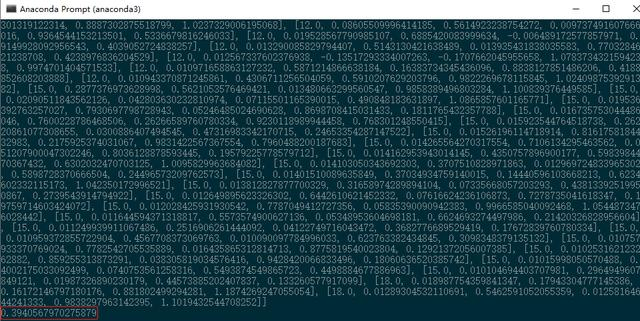```// MainActivity.java L41 protected long[] inputShape = new long[]{1, 3, 300, 300}; protected float[] inputMean = new float[]{0.5f, 0.5f, 0.5f}; protected float[] inputStd = new float[]{0.5f, 0.5f, 0.5f};
// Predictor.java L214 // Set input shape Tensor inputTensor = getInput(0); inputTensor.resize(inputShape);
// Predictor.java L258 inputTensor.setData(inputData);
// Predictor.java L303 float rawLeft = outputTensor.getFloatData()[i + 2]; float rawTop = outputTensor.getFloatData()[i + 3]; float rawRight = outputTensor.getFloatData()[i + 4]; float rawBottom = outputTensor.getFloatData()[i + 5];```

`// main.dart L127 var ratioW = sizeRed.width / 300; var ratioH = sizeRed.height / 300;`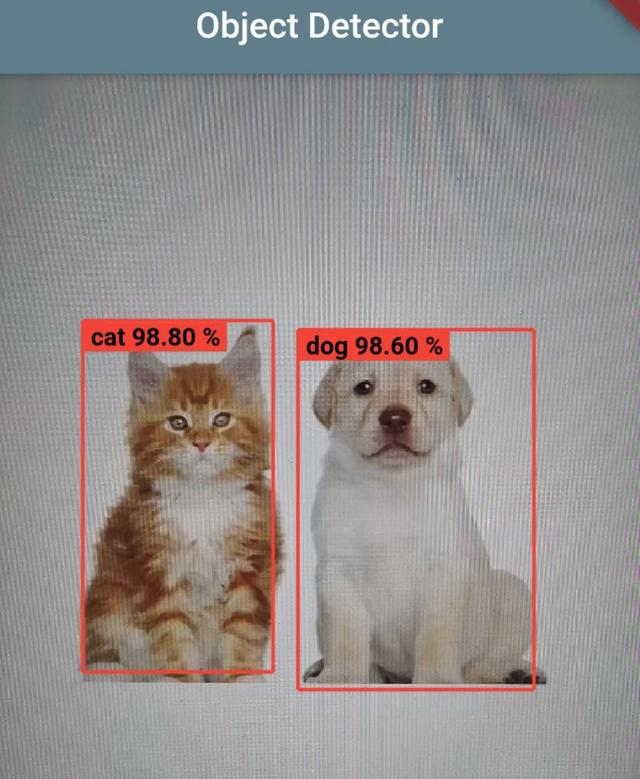## 参考链接：

• AI Studio: https://aistudio.baidu.com/
• Real-time Object Detector:https://github.com/KernelErr/realtime-object-detector

GitHub: